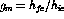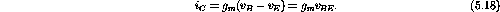Next: The Common Emitter Amplifier Up: Bipolar Junction Transistors Previous: Ideal and Perfect Bipolar

## Transconductance Model

The transconductance model provides an alternative description of transistor operation. The forward transconductance, which has units of inverse resistance, is defined as. Using the perfect transistor model we can writeSince the transconductance is used to describe field effect transistors, it is sometimes convenient to apply the same parameter to bipolar transistors.

We now turn to the description of some simple amplifiers that use a single bipolar transistor. Our goal will be to estimate the voltage gains, current gains, input impedances, output impedances and corner frequencies of the amplifiers. The characteristics of a perfect amplifier are as follows:

• infinite input impedance (the amplifier draws no current),
• zero output impedance,
• infinite voltage and current gain, and
• stable, linear, etc.

Doug Gingrich
Tue Jul 13 16:55:15 EDT 1999